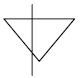Name:    3rd Grade Math Proficient Test 4

Multiple Choice
Identify the choice that best completes the statement or answers the question.

1.

Computation – Fractions – RIT 211 – 220

Write 6/12 in simplest form.
 a. 1/2 d. 1/6 b. 12/24 e. 1/6 c. 2/4

2.

2/10 + 4/10 =
 a. 3/10 d. 6/20 b. 3/5 e. 6/10 c. 2/5

3.

1 7/8 + 3 3/4 =
 a. 4 10/12 d. 5 3/4 b. 4 7/8 e. 45/8 c. 5 5/8

4.

Multiply and simplify.
5 x 1/3 =
 a. 15/9 d. 1 2/3 b. 1 6/9 e. 14/3 c. 16/3

5.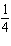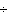=
 a. 3/7 d. 1 b. 11/12 e. 3/8 c. 2/12

6.

3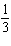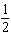=
 a. 6 2/3 d. 22/3 b. 3 5/6 e. 6 1/2 c. 2 5/6

7.

Order each fraction from least to greatest.
1/2, 1/3, 5/6, 2/3, 1/6
 a. 5/6, 1/6, 2/3, 1/3, 1/2 d. 1/6, 1/3, 1/2, 2/3, 5/6 b. 5/6, 2/3, 1/2, 1/3, 1/ e. 1/2, 1/3, 5/6, 2/3, 1/6 c. 5/6, 2/3, 1/6, 1/3, 1/

8.

Computation – Integers – RIT 211 – 220

Which statement is true?
a = 12
b = 21

 a.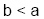d.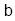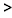b.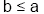e.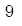c.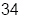9.

–6 + (-7) =
 a. +13 d. 1 b. –1 e. –13 c. 42

10.

5 + (-15) =
 a. 10 d. –10 b. –20 e. –75 c. 20

11.

6 + (-2) + 3 + (-5) =
 a. 2 d. 6 b. 16 e. -2 c. –16

12.

Multiply these two integers.
(-8) (6) =
 a. 14 d. –48 b. –14 e. –2 c. 48

13.

- 246 =
 a. 4 d. –18 b. –4 e. -30 c. 18

14.

Computation – Whole Numbers – RIT 211 – 220

426 = ____
 a. 6 x 7 = 39 d. 7 < 42 b. 42 > 6 e. 6 x 7 = 42 c. 7 -  6 = 1

15.

3,0476 = ____
 a. 3,647 d. 507 R5 b. 500 R7 e. 57 R5 c. 18,282

16.

When you multiply any number by 10 you add a ______ to that number.
 a. 10 d. 00 b. 0 e. decimal c. 1

17.

8,53276 = ___
 a. 8,456 d. 101 R20 b. 1,120 e. 112 R20 c. 8,608

18.

Geometry – Shapes and Figures – RIT 191 – 200

Find the sphere.
 a.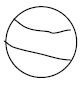d.b.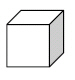e.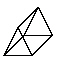c.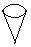19.Name the figure.
 a. cylinder d. sphere b. cone e. pyramid c. cube

20.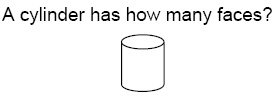a. 2 d. 4 b. 0 e. 1 c. 3

21.

Geometry – Symmetry and Transformations – RIT 191 – 200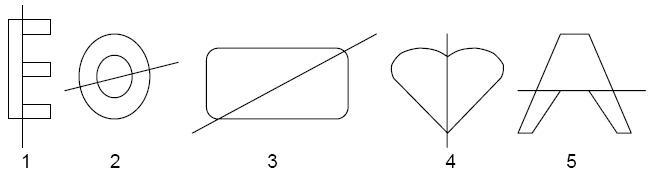Which figure or figures show(s) a line of symmetry?
 a. 2, 3, and 4 d. 2 and 4 b. 2 only e. 3, 4, and 5 c. 1 and 2

22.

Geometry – Geometric Properties & Terminology – RIT 191 – 200

Which letter is in the middle?
A B C D E F G
 a. E d. G b. C e. D c. A

23.

Which figure is a right angle?
 a.b.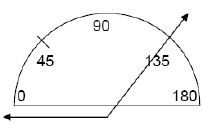c.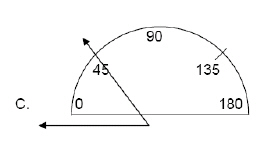d.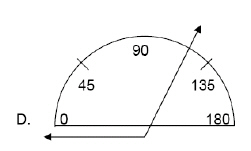e.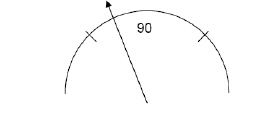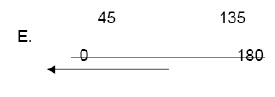24.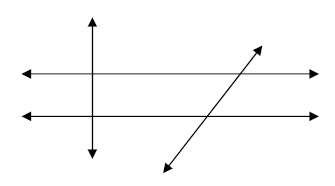How many angles are right angles?
 a. 2 d. 16 b. 8 e. 6 c. 4

25.

How many sets of parallel lines are in the figure?a. 1 d. 5 b. 0 e. 3 c. 2

26.

Which figure shows a diagonal line?
 a.d.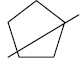b.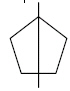e.c.